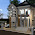## Tuesday, April 3, 2018

Pile caps are concrete mats that rests on piles with adequate rigidity to transfer the column loads to the piles. Piles are provided as alternatives to shallow foundations when a firm and stable soil to carry column load is too deep below the surface, or when high lateral load is anticipated. More often than not, pile caps are usually so rigid that they make the entire group of piles to behave like one unit.

EN 1992-1-1:2004 permits us to use strut and tie models to analyse structures where non-linear strain distribution exists (e.g. pile caps, deep beams and corbels).

In strut and tie models, trusses are used with the following components:
• Struts (concrete)
• Ties (reinforcement)
• Nodes (intersections of struts and ties)

Eurocode 2 gives guidance for each of these.

Design Example
A 500mm x 500mm column is carrying an ultimate limit state load of 2140 kN. We are to design the pile cap using the following data;

Grade of concrete fck = 30 N/mm2
Fyk = 500 N/mm2
Concrete cover = 75mm
Spacing of pile = 1800mm
Diameter of piles = 600mm
Solution
The strut and tie model is as given below;

Angle of inclination of strut γ = tan-1 (1100/900) = 50.710°
cos  γ = 0.633
sin γ = 0.7739

Force in strut Fs = 1070/sinγ = 1382.6075 kN

Force in tie Ft = 1382.6075 cos γ = 875.15 KN

Area of tension steel required As = Ft/0.87Fyk = (875.15 × 1000) / (0.87 × 500) = 2011.8 mm2
Provide 7H20 @ 150 c/c (Asprov = 2189 mm2 )
Asmin = 0.13bh/100 = 0.0013 × 900 × 1200 = 1404 mm2

Since pile spacing is less than three times pile diameter, the bars may be spread uniformly across the cap.

Check for Shear
Consider the critical section for shear to be located at 20% of the pile diameter inside the pile cap.

Distance of this section from the column face;
av = 0.5(Spacing between piles - width of column) - 0.3(pile diameter)
av = 0.5(1800 - 500) - 0.3(600) = 470 mm

Length of corresponding perimeter for punching shear
u = 2(900 + 1440) = 4680mm

Perimeter of pile cap = 2(900 + 2700) = 7200mm

Since the perimeter of the pile cap is less than 2u, normal shear extending across the full width of the pile cap is more critical than punching shear.

The contribution of the column load to the shear force may be reduced by applying a factor β = av/2d, where 0.5d ≤ a≤ 2d

But a little consideration will show that av(470 mm) < 0.5d(550 mm), therefore, take av as 0.5d (550)
Therefore β = 550 / 2(1100) = 0.25

v = βV/bd

V = 1070 KN + (Self weight of pile cap/2)
Self weight of pile cap = 1.35(25 × 2.7 × 0.9 × 1.2) = 98.415 KN

v = (0.25 × 1119.2 × 1000)/(2700 × 1100) = 0.0942 N/mm2

VRd,c = [CRd,c.k.(100ρ1 fck)(1/3) + k1cp] ≥ (Vmin + k1cp)

CRd,c = 0.18/γc = 0.18/1.5 = 0.12
k = 1 + √(200/d) = 1 + √(200/1100) = 1.426 > 2.0, therefore, k = 1.426
Vmin = 0.035k(3/2) fck0.5
Vmin = 0.035 × (1.426)1.5 × 300.5 = 0.326 N/mm2
ρ1 = As/bd = 2185/(2700 × 1100) = 0.0007356 > 0.02; Therefore take 0.02

VRd,c = [0.12 × 1.426 (100 × 0.0007356 × 30)(1/3)]  = 0.2227  N/mm2

Shear is ok.

Punching Shear Along Column Perimeter
V = 2140 KN

v = V/ud
v = (2140 × 1000)/(2000 × 1100) = 0.972 N/mm2

VRd,max = 0.2(1 -  fck/250)fck
VRd,max = 0.2 (1 - 30/250)30 = 5.28 N/mm2
This shows that the punching shear around column perimeter is ok.

Extra
This pile cap has been modelled on Staad Pro and the results obtained are given below.The set back in the model is the piles were modelled as columns that could sway under the load. What do you think?

Thank you for visiting Structville today and God bless. Kindly share with your folks.

1.2.